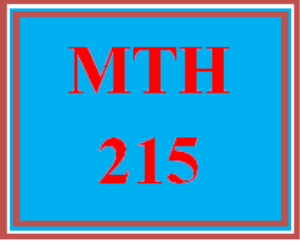# MTH 215 Week 4 MyMathLab® Study Plan for Week 4 Checkpoint

PLDZ-5079 In Stock
\$ 8.00 USD
Description

MTH 215 Week 4 MyMathLab® Study Plan for Week 4 Checkpoint

https://uopcourses.com/category/mth-215/

# MyMathLab® Study Plan for Week 4 Checkpoint

Please use the following link to launch the content: External Content Launch

• 8.A Growth: Linear versus Exponential

• Decide if a statement involving linear and exponential growth makes sense.

• Distinguish between linear and exponential growth or decay.

• Solve applications involving linear and exponential growth.

• 9.A Functions: The Building Blocks of Mathematical Models

• Determine if two variables are related in a way that can be described as a function.

• Describe the relationship between two variables.

• Graph and describe functions.

• 9.B Linear Modeling

• Decide if a statement involving linear models makes sense.

• Analyze characteristics of linear graphs.

• Solve applications involving linear functions.

• Graph linear equations.

• 9.C Exponential Modeling

• Decide if a statement involving exponential models makes sense.

• Brief Review – Logarithms

• Solve applications of exponential growth and decay.

Decide whether the following statement makes sense? (or is clearly? true) or does not make sense? (or is clearly? false). Explain your reasoning.

Money in a bank account earning compound interest at an annual percentage rate of? 3% is an example of exponential growth.

The statement does not makes sense because the money in the account grows by the same absolute? amount, which is an example of linear growth.

The statement makes sense because the money in the account grows by the same? percentage, which is an example of exponential growth.

The statement makes sense because the money in the account grows by the same absolute? amount, which is an example of exponential growth.

The statement does not make sense because the money in the account grows by the same? percentage, which is an example of linear growth.

Recent Reviews Write a Review
0 0 0 0 reviews